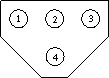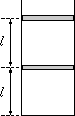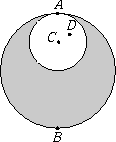Középiskolai Matematikai és Fizikai Lapok
Informatika rovattal
 Már regisztráltál? Új vendég vagy?

# Exercises and problems in Physics December 1997

## New experimental problem:

m. 193. Determine the directions in which pictures of objects can be seen through a binocle and the magnifications. Does the magnification depend on the distance of the object?

## New exercises:

FGy. 3110. Two candles of identical lengths and different thicknesses were lit at Christmas. Half of the thin candle and one third of the thick candle could be seen to burn down in 15 minutes. How long after the candles had been lit was the ratio of their lengths 4?FGy. 3111. The tramway control lights show red when lights 1, 2 and 3 are on, and it shows green when lights 2 and 4 are on. What circuit makes it possible to turn the lights from green to red or from red to green with a single switching?

FGy. 3112. Hanging a 3 dkg body on each of a 12 cm and a 9 cm spring makes both springs extend by one third of their original lengths. Half of the long spring and two thirds of the short spring are cut off, therefore fastening the ends of the remaining springs together gives a 9 cm-long spring. At what frequency will a 6 dkg body oscillate on this spring?FGy. 3113. There are two pistons of negligible thickness and of mass m each in a vertically standing cylindrical container as shown in the figure. The upper piston is slowly pushed down until it arrives at the initial position of the lower piston. Where is the lower piston at this moment? The area of the cross-section of the cylinder is A, the atmospheric pressure is p0. (Both pistons can move without friction; consider the temperature as constant.)

## New problems:FF. 3114. Astronauts land on an asteroid of radius R and homogeneous mass distribution. A physicist astronaut makes a simple pendulum and measures its period to be 1 second. As precious ores can be found in the asteroid, a sphere of radius R/2 is exploited and removed from the asteroid (see the figure). What will the period of the pendulum be in points A, B, C and D shown in the figure?

FF. 3115. The bottom one of two horizontal metal plates of area A and mass m is fixed and the top one hangs on a spring of force constant D. Connecting voltage U between the plates the equilibrium distance between the plates is d. What is the frequency of the small amplitude oscillations that the top plate can do when

a) the battery is switched off,
b) the battery remains switched on during the oscillations of the plate?

FF. 3116. On 6 November, 1997, Venus could be seen at a maximum deviation of 47o East of the Sun. The previous time Venus was in such a position was on 1 April, 1996, when it could be seen at an angle of 46o from the Sun. (The reason for the slight difference between the angles is that the planets move on elliptical orbits.) Determine the period of Venus. When will Venus next be at its maximum deviation to the West?

FF. 3117. An airplane moves at a uniform speed in a horizontal plane. What is the curve of its track if there is a point that the sound of the plane reaches at the same time from any point of the track?